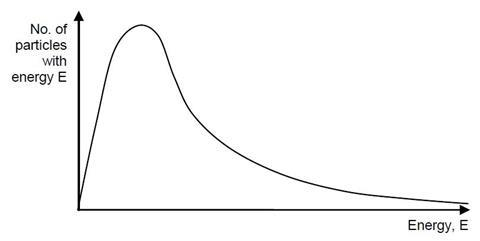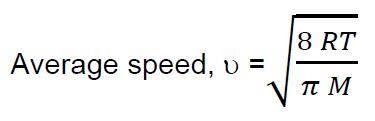Allow students the opportunity to write about science, and share their critical thoughts on computer simulations in their education

The students are contacted to write a review on the use of computer simulations in sixth form chemistry for the student chemistry magazine “The Mole”.

## Pre-lab questions

(Remember to give full references for any information beyond A-level that you find out)

1. The Maxwell-Boltzmann distribution is used to describe the energies of particles in a gas at a certain point in time. It applies only to ideal gases. Describe the key assumptions that distinguish an ideal gas from a real gas.

2. The diagram opposite represents a Maxwell-Boltzmann distribution curve for the particles in a sample of gas at a certain temperature, T;a) Indicate on the curve above an approximate value for;

i. the most probable energy of a gas particle

ii. the average energy of a gas particle in this system

b) The Kinetic Theory of Gases describes equations for calculating particle speeds based on their energies. Since there is a distribution of particle speeds in the system, scientists use different measures for the particle speed. Two such measures are the average speed of a gas particle and the most probable speed of a gas particle.

The average speed of a gas particle in a system is given by the equation;i. Define all the symbols in this equation including UNITS

ii. A similar equation allows the most probable speed of a gas particle to be calculated. Define this equation.

3. In chemistry, many functions can be represented by a straight line graph of the type y = mx + c where; ‘x’ and ‘y’ are the coordinates of the points that satisfy the function ‘m’ is the gradient of the straight line graph, and ‘c’ is the ‘y intercept’ of the straight line graph

In the equation for the average speed of a gas particle given in question 2 b), if you plotted a graph of √𝑇 (x-axis) against the average speed, υ (y-axis), what value would the gradient of your graph be equal to?

## Each group will need;

Or

At the time of going to print, the URL for the PhET Gas Properties Simulation is;